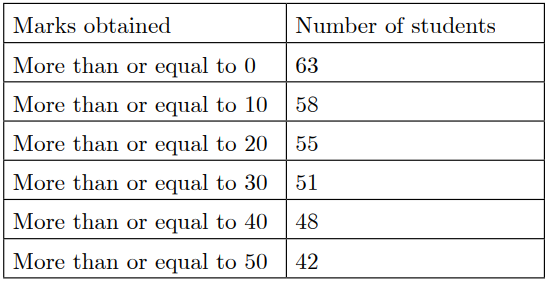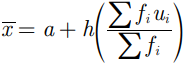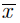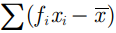Saturday, December 4, 2021
Home > CBSE Class 10 > Extra MCQ Questions for Class 10 Maths Chapter 14 Statistics Mean Median Mode

# Extra MCQ Questions for Class 10 Maths Chapter 14 Statistics Mean Median ModeHi students, Welcome to AMBiPi (Amans Maths Blogs). In this article, you will get Extra MCQ Questions for Class 10 Maths Chapter 14 Statistics Mean Median Mode. You can download this PDF and save it in your mobile device or laptop etc.

Extra MCQ Questions for Class 10 Maths Statistics Question No 31:

For the following distribution: Find the frequency of class 30-40.Option A : 3

Option B : 4

Option C : 48

Option D : 51

Option A : 3

Extra MCQ Questions for Class 10 Maths Statistics Question No 32:

In the following distribution, the times, in seconds, taken by 150 athletes to run a 110 m hurdle race are given. The number of athletes who completed the race in less than 14.6 second is :Option A : 11

Option B : 71

Option C : 82

Option D : 130

Option C : 82

Extra MCQ Questions for Class 10 Maths Statistics Question No 33:

For the following distribution, find the difference of the upper limit of the median class and the lower limit of the modal class.Option A : 0

Option B : 19

Option C : 20

Option D : 38

Option C : 20

Extra MCQ Questions for Class 10 Maths Statistics Question No 34:

For the following distribution: Find the modal class.Option A : 10-20

Option B : 20-30

Option C : 30-40

Option D : 50-60

Option C : 30-40

Extra MCQ Questions for Class 10 Maths Statistics Question No 35:

In the formula, for finding the mean of grouped frequency distribution, ui is equal to

Option A : (xi + a) / h

Option B : h(xi – a)

Option C : (xi – a) / h

Option D : (a – xi) / h

Option C : (xi – a) / h

Extra MCQ Questions for Class 10 Maths Statistics Question No 36:

If xi‘s are the mid points of the class intervals of grouped data, fi’s are the corresponding frequencies andis the mean, then the value ofis

Option A : 0

Option B : -1

Option C : 1

Option D : 2

Option A : 0

Extra MCQ Questions for Class 10 Maths Statistics Question No 37:

If the mean of a, b, c is M and (ab + bc + ca) = 0, then the mean of a2, b2 and c2 is kM2, then find k.

Option A : 3

Option B : 9

Option C : 6

Option D : 4

Option A : 3

Extra MCQ Questions for Class 10 Maths Statistics Question No 38:

If X, Y and Z denote mean, median and mode of the data such that X : Y = 9 : 8, then find the ratio of Y : Z.

Option A : 3 : 4

Option B : 4 : 9

Option C : 4 : 3

Option D : 2 : 5

Option C : 4 : 3

Extra MCQ Questions for Class 10 Maths Statistics Question No 39:

If the mean of the squares of first n natural numbers is 105, then the median of the first n natural numbers is

Option A : 8

Option B : 9

Option C : 10

Option D : 11

Option B : 9

Extra MCQ Questions for Class 10 Maths Statistics Question No 40:

Observations of some data are x/5, x, x/3, 2x/3, x/4, 2x/5 and 3x/4, where x > 0. If the median of the data is 4, then the value of x is

Option A : 5

Option B : 15

Option C : 9

Option D : 10

Option D : 10

CBSE Class 10 Maths Chapterwise MCQs
1 : Real Numbers MCQ
| 1 to 10 | 11 to 20 | 21 to 30 | 31 to 40 | 41 to 50
2 : Polynomials MCQ
| 1 to 10 | 11 to 20 | 21 to 30 | 31 to 40 | 41 to 50
3 : Linear Equations MCQ
| 1 to 10 | 11 to 20 | 21 to 30 | 31 to 40 | 41 to 50
| 1 to 10 | 11 to 20 | 21 to 30 | 31 to 40 | 41 to 50
5 : Arithmetic Progression MCQ
| 1 to 10 | 11 to 20 | 21 to 30 | 31 to 40 | 41 to 50
6 : Triangles MCQ
| 1 to 10 | 11 to 20 | 21 to 30 | 31 to 40 | 41 to 50
7 : Coordinate Geometry MCQ
| 1 to 10 | 11 to 20 | 21 to 30 | 31 to 40 | 41 to 50
8 : Introduction to Trigonometry MCQ
| 1 to 10 | 11 to 20 | 21 to 30 | 31 to 40 | 41 to 50
9 : Application of Trigonometry MCQ
| 1 to 10 | 11 to 20 | 21 to 30 | 31 to 40 | 41 to 50
10 : Circles MCQ
| 1 to 10 | 11 to 20 | 21 to 30 | 31 to 40 | 41 to 50
11 : Constructions MCQ
| 1 to 10 | 11 to 20
12 : Area Related to Circles MCQ
| 1 to 10 | 11 to 20 | 21 to 30 | 31 to 40 | 41 to 50
13 : Surface Area & Volume MCQ
| 1 to 10 | 11 to 20 | 21 to 30 | 31 to 40 | 41 to 50
14 : Statistics MCQ
| 1 to 10 | 11 to 20 | 21 to 30 | 31 to 40 | 41 to 50
15 : Probability MCQ
| 1 to 10 | 11 to 20 | 21 to 30 | 31 to 40 | 41 to 50
16 : Previous Year Maths MCQs from CBSE Board Class 10
| 1 to 100
AMBiPi
error: Content is protected !!